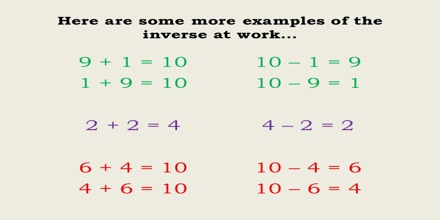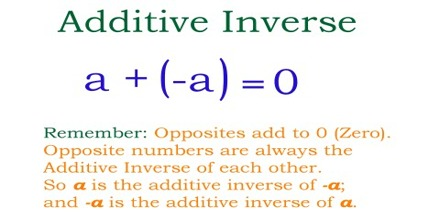Inverse relationship of Addition and Subtraction

The word ‘inverse’ means reverse in direction or position. It comes from the Latin word ‘inversus,’ which means to turn upside down or inside out. In mathematics, an inverse operation is an operation that undoes what was done by the previous operation.There is an inverse relationship between addition and subtraction.

If a math fact is considered, for example 3 + 7 = 10. Then the following are also true:

10 – 3 = 7

10 – 7 = 3

Similar relationships exist for subtraction, for example 10 – 3 = 7. Then the following are also true:

3 + 7 = 10

7 + 3 = 10

The reason for this is that we are dealing with an equation. An equation is balanced or the same on either side of the equals (=) sign. If exactly the same thing is done to both sides of the equation, it will still be balanced or equal.

In the example above we start with the equation 3 + 7 = 10

Subtract the same number from both sides 3 + 7 – 3 = 10 – 3

On the left side the 3 and -3 produce 0 which leaves 7 = 10 – 3

Turning the equation around to be in more normal form 10 – 3 = 7